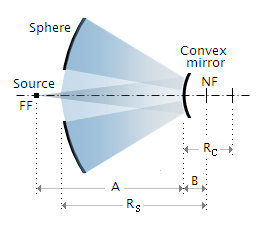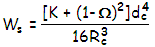telescopeѲptics.net          ▪▪▪▪                                             CONTENTS

# 4.8.5. Hindle sphere test

Testing convex surfaces is complicated by the absence of real focus. An alternative with two-mirror systems is finishing the primary first, and use it to test (null) the secondary in collimated light. Another possibility is to use centrally perforated spherical mirror, separated from the convex surface under test so that its center of curvature coincides with the near focus of the convex surface's conic. By placing light source at the far focus of the convex surface, with light traveling through the perforation on the sphere to the convex surface, reflecting to the sphere and back to the surface, which focuses it back toward the source point, there is no aberration induced if the convex surface is accurately made. This setup, which makes possible to null hyperboloidal convex surfaces, is called Hindle sphere test (FIG. 56).FIGURE 56: The Hindle sphere test setup: light source is at the far focus (FF) of the convex surface of the radius of curvature RC and eccentricity ε, and Hindle sphere center of curvature coincides with its near focus (NF). Far focus is at a distance A=RC/(1-ε) from convex surface, and the radius of curvature (RS) of the Hindle sphere is a sum of the mirror separation and near focus (NF) distance (absolute values), with the latter given by B=RC/(1+ε). Thus, the mirror separation equals RS-B. The only requirement for the sphere radius of curvature RS is to be sufficiently smaller than the sum of near and far focus distance to make the final focus accessible. Needed minimum sphere diameter is larger than the effective test surface diameter by a factor of RS/B. Clearly, Hindle test is limited to surfaces with usable far focus, which eliminates sphere (ε=0, near and far focus coinciding), prolate ellipsoids (1>ε>0, near and far foci on the same, concave side of the surface), paraboloid (ε=1, far focus at infinity) and hyperboloids close enough to a paraboloid to result in an impractically distant far focus.

Test accuracy depends on the proper placement of the two surfaces. The source distance (A, equal to the far focus distance) is very forgiving in this respect, due to the aberration created on the test surface by inaccurate source placement nearly offsetting in its two reflections. This error is not likely to become worth noticing for typical surfaces tested by amateurs before axial source displacement runs into inches. The error at the testing sphere remains negligible.

The Hindle setup is much more sensitive to the errors in mirror separation. It rapidly produces spherical aberration, practically all generated by the second reflection off the convex surface. The reason is that the compensatory mechanism doesn't work anymore: by moving closer to, or farther away from the sphere, the convex surface changes its distance with respect to sphere's (imaginary) focus, which represents the surface's object. In other words, its object location no longer coincides with its near (conic) focus, and it results in aberration, which is this time not offset by practically non-existent aberration generated by the first reflection.

Using paraxial approximation to find out the change in location of the image formed by the sphere caused by the change in in mirror separation Δ, we find that the shift with respect to its proper position is ; in other words, given reduction in separation will result in the sphere forming its imaginary focus as much farther away, and vice versa. This means that the new object location for the convex surface is shifted twice as much, or 2Δ, away from its near focus, resulting - through the change of ray height at the surface and its magnification - in the introduction of spherical aberration.

For given mirror separation error Δ, the resulting P-V wavefront error at the best focus is found from Eq. 9.1 and Eq. 7, as:where dC is the effective convex surface semi-diameter, K=-ε2 is the surface conic and Ω=RC/(B+2Δ), the reciprocal of the object distance in units of the radius of curvature (both, RC and B are numerically positive). Obviously, the sign of Δ matters little, and the wavefront error is nearly identical in magnitude for relatively small deviations from the exact separation, whether the separation is smaller or larger than what it needs to be (for relatively large deviations from proper separation, change in the height of marginal ray on the second reflection from test surface, which diminishes with the increase in separation, and increases with its reduction, becomes significant, resulting in the wavefront error significantly larger with the latter for the same absolute change in separation). In general, larger than proper separation induces over-correction, and smaller under-correction.

A Hindle test setup in OSLO, with the following parameters needed for error calculation, K=-2.25 (ε=1.5), dC=25mm, RC=300mm and B=120mm, (the rest of parameters are A=600mm, RS=620mm, with the effective sphere semi-diameter of 128mm, and mirror separation of 500mm), with Ω=2.459 for Δ=1mm, generates 0.2 wave P-V wavefront error of lower-order spherical aberration (the higher-order term is negligible).

This is already unacceptable for higher standards; maximum acceptable error is probably half as large, but preferably not more than 1/20 wave P-V. Since the P-V error is proportional to the spacing error Δ, this would put mirror separation tolerance for this setup at ±0.25mm, or 0.5mm max.

While it seems to be a tight tolerance, it is fairly easy to control using the side-effect of the spacing error, namely the change in convex surface magnification. In the above setup, a 1mm change in spacing resulted in the shift of the final focus by over 40mm: a magnitude of change easy to detect and control by smoothly adjusting the test surface location (keeping the final focus within ±10mm from its ideal location for this setup would ensure 1/20 wave P-V or better accuracy). This implies that Hindle sphere test does require some sort of smoothly moving platform for axial adjustment of the test surface location.

An alternative for determining the conic of any convex surface is simple interferometric test against an accurate concave sphere of similar radius of curvature as the surface tested. If the radius of a sphere is known, the sagitta (depth) differential between two surfaces can be calculated using Eq. 4.6. Based on the differential, the appropriate interference pattern of concentric circles can be determined, that should be produced when the two surfaces are in contact, against each other. The advantage of this test type is in its direct visual interface, which also gives clear indication of what type of deviation - if any - is present. It requires, however, that the test surface is made in a substrate of satisfactory homogeneity (near-optical-quality glass), as well as use of near-monochromatic light.

Yet another alternative is to null convex surface through its back, as a concave surface. Since there is a double refraction taking place as light travels from the source to the test surface and back to the null, it is necessary to compute needed element separation. This type of test also can use accessory optical elements, such as spherical mirror of known curvature radius, or lens; it can also be done by putting appropriate curve on the back side. For hyperboloidal surfaces, none of it is required.